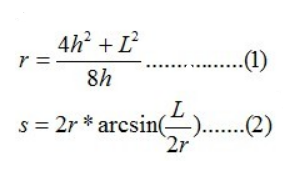# POJ 1905 - Expanding Rods

## 解题思路

• 蓝色为杆弯曲前，长度为L
• 红色为杆弯曲后，长度为s
• h是所求• （1）角度→弧度公式 `θr = 1/2*s`
• （2）三角函数公式 `sinθ= 1/2*L/r`
• （3）勾股定理 `r^2 – (r – h)^2 = (1/2*L)^2`• 要求（1）式的h，唯有先求r
• 但是由于（2）式是三角函数式，直接求r比较困难

## AC 源码

``````//Memory Time
//244K   0MS

#include<iostream>
#include<math.h>
#include<iomanip>
using namespace std;

const double esp=1e-5;   //最低精度限制

int main(void)
{
double L,n,c,s;   //L:杆长 ，n:温度改变度 ， c:热力系数  ，s:延展后的杆长（弧长）
double h;    //延展后的杆中心 到 延展前杆中心的距离
double r;   //s所在圆的半径

while(cin>>L>>n>>c)
{
if(L<0 && n<0 && c<0)
break;

double low=0.0;    //下界
double high=0.5*L; //  0 <= h < 1/2L   (1/2L并不是h的最小上界，这里做一个范围扩展是为了方便处理数据)

double mid;
s=(1+n*c)*L;
while(high-low>esp)  //由于都是double，不能用low<high，否则会陷入死循环
{                    //必须限制low与high的精度差
mid=(low+high)/2;
r=(4*mid*mid+L*L)/(8*mid);

if( 2*r*asin(L/(2*r)) < s )     //h偏小
low=mid;
else       //h偏大
high=mid;
}
h=mid;

cout<<fixed<<setprecision(3)<<h<<endl;
}
return 0;
}``````

目录